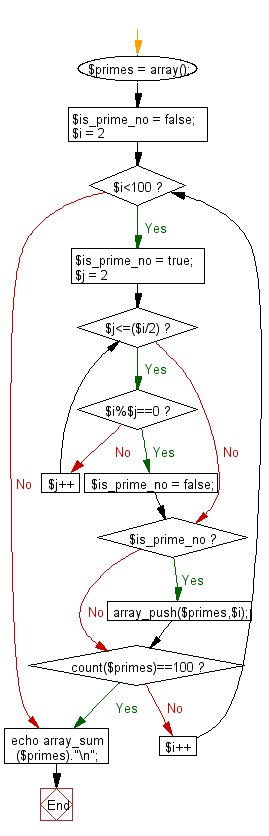﻿ PHP Exercise: Compute sum of the prime numbers less than 100 - w3resource# PHP Exercises: Compute sum of the prime numbers less than 100

## PHP: Exercise-37 with Solution

Write a PHP program to compute the sum of the prime numbers less than 100.

Note: There are 25 prime numbers are there in less than 100.
2, 3, 5, 7, 11, 13, 17, 19, 23, 29, 31, 37, 41, 43, 47, 53, 59, 61, 67, 71, 73, 79, 83, 89, 97 and sum of all these numbers is 1060.

Sample Solution: -

PHP Code:

``````<?php
\$primes = array();
\$is_prime_no = false;
for (\$i = 2; \$i<100; \$i++) {
\$is_prime_no = true;
for (\$j = 2; \$j<=(\$i/2); \$j++) {
if (\$i%\$j==0) {
\$is_prime_no = false;
break;
}
}
if (\$is_prime_no) {
array_push(\$primes,\$i);
}
if (count(\$primes)==100) {
break;
}
}
echo array_sum(\$primes)."\n";
?>
```
```

Sample Output:

```1060
```

Flowchart:PHP Code Editor:

Have another way to solve this solution? Contribute your code (and comments) through Disqus.

What is the difficulty level of this exercise?

﻿

## PHP: Tips of the Day

How do I strip all spaces out of a string in PHP?

Do you just mean spaces or all whitespace?

For just spaces, use str_replace:

`\$string = str_replace(' ', '', \$string);`

For all whitespace (including tabs and line ends), use preg_replace:

`\$string = preg_replace('/\s+/', '', \$string);`

Ref : https://bit.ly/2Cnb3QZ